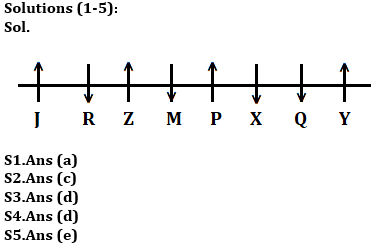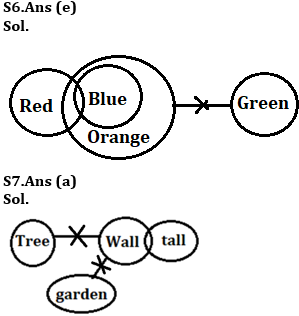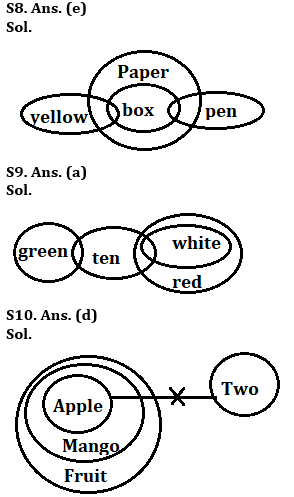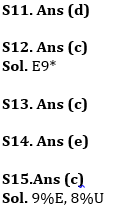Latest Banking jobs   »   Reasoning Ability Quiz For ESIC- UDC,...

# Reasoning Ability Quiz For ESIC- UDC, Steno, MTS Prelims 2022- 18th January

Directions (1-5): Study the information carefully and answer the questions given below.
Eight persons are sitting in a row some of them facing north and some of them facing south. M sits third to the right of J, who sits one of the extreme ends of the row. Six persons sit to the right of Q. Z sits fourth to the right of Q. Immediate neighbor of Z face same direction to each other’s. Both X and P sits immediate right to each other’s. One person sits between P and Z. P sits third to the left of R. R is not immediate neighbor of Q and M. Not more than four persons face south direction. P sits third to the left of Y. M does not face North.

Q1.Who among the following person sits immediate left of Z?
(a) R
(b) M
(c) J
(d) X
(e) None of these

Q2. How many persons sits between Z and Q?
(a) Two
(b) One
(c) Three
(d) Four
(e) None

Q3. How many persons face north?
(a) Six
(b) Five
(c) Three
(d) Four
(e) Two

Q4. Four of the following five are alike in certain way based from a group, find the one that does not belong to that group?
(a) X
(b) Q
(c) M
(d) Y
(e) R

Q5. Who among the following sits immediate right of Y?
(a) Q
(b) X
(c) Z
(d) M
(e) No one

Directions (6-10): In each of the questions below are given some statements followed by some Conclusions. You have to take the given statements to be true even, if they seem to be at variance from commonly known facts. Read all the conclusions and then decide which of the given conclusions logically follows from the given statements disregarding commonly known facts.
(a) If only conclusion I follows.
(b) If only conclusion II follows.
(c) If either conclusion I or II follows.
(d) If neither conclusion I nor II follows.
(e) If both conclusions I and II follow.

Q6. Statements:
Some Red are Blue
All blue are Orange
No orange is green
Conclusions:
I: Some blue are not green
II: All red can never be Green

Q7. Statements:
No tree is wall
Some wall is tall
No wall is garden
Conclusions:
I: All tree can be tall
II: Some tall are garden

Q8. Statements:
All box is paper
Some box is Pen
Only a few yellow are box
Conclusions:
I. All yellow can be paper
II. All pen can be box

Q9. Statements:
Some Green are ten
Some ten are white
All white are Red
Conclusions:
I. Some green are white is possibility
II. No Green is white

Q10. Statements:
All apple are mango
All mango are Fruit
No apple are two
Conclusions:
I. All two can never be Mango
II. All mango are apple

Directions (11-15): Study the following alphanumeric series carefully and answer the questions given below:

Q @ 7 R T 8 9 % E 9 * 3 4 \$ S 5 V B S * 7 B \$ * G 2 8 % U 2 4 # F

Q11. Which of the following element is 22nd from right end of the series?
(a) *
(b) 4
(c) 9
(d) 3
(e) None of these

Q12. How many such numbers are there which is preceded by alphabets and followed by symbols?
(a) Two
(b) Four
(c) One
(d) Three
(e) None

Q13.How many consonants are between the element which is 9 from the left end and 12th from the right end?
(a) Five
(b) Three
(c) Four
(d) One
(e) None

Q14.If all numbers are eliminated from the series then, which of the following element is 9th from left end of the series?
(a) \$
(b) 5
(c) 4
(d) V
(e) None of these

Q15. How many such symbols are there which is preceded by number and followed by vowels?
(a) Three
(b) None
(c) Two
(d) Four
(e) One

Solutions#### Congratulations!